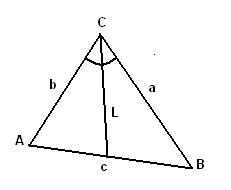homechevron_rightStudychevron_rightMathchevron_rightGeometry

# Angle bisector of a triangle

This online calculator computes the length of angle bisector given the lengths of triangle edges

### This page exists due to the efforts of the following people:

#### MicheleThis online calculator computes the length of the angle bisector given the lengths of triangle edges (see the picture). Triangle vertices are usually named A, B, and C. Triangle edges - a, b, c, where the letter denotes opposite vertex. That is the edge between A and B is named c, between A and C - b, between B and C - a.
The length of С's angle bisector shown on picture is:#### Angle bisector of a triangle

Digits after the decimal point: 2
Length of angle bisector

URL copied to clipboard

#### Similar calculatorsPLANETCALC, Angle bisector of a triangle